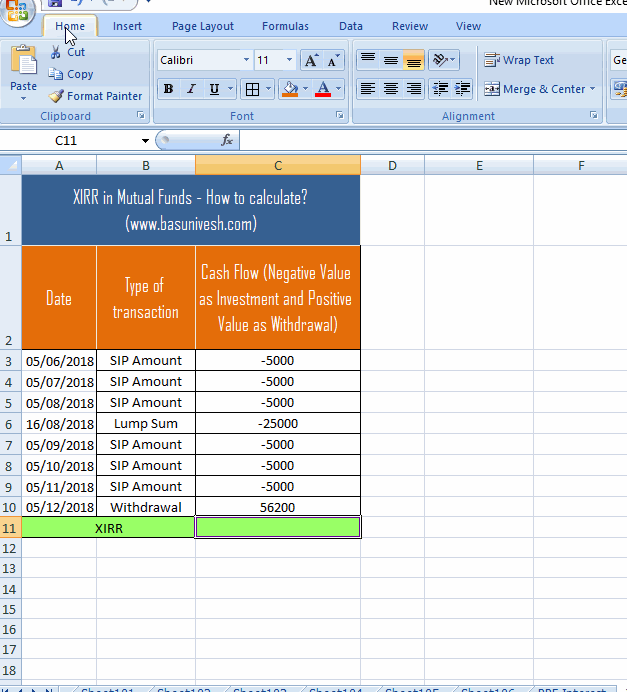# XIRR in Mutual Funds – What is this and how to calculate?

What is XIRR in Mutual Funds? How to calculate it? Many of the Mutual Fund Investors might have come across the word XIRR. But for the majority of them, it is hard to digest of what it is. Hence, let us simplify it.

## What is CAGR?

There is a huge misconception in understanding the difference between CAGR and XIRR. Hence, first let us understand the meaning of CAGR. CAGR stands for “compounded annualized growth rate”. This means your every investment are annualized to arrive at CAGR. It basically points to point return.

The formula used to calculate this as below.

CAGR = ((Ending Amount (FV)/Beginning Amount(PV))^(1/No. of years(N))) – 1

If we assume that we invested Rs.10,000 today and after 5 years, the value of that is Rs.15,000, then the average rate at which investment has compounded year after year is as below.

8.44%=((15,000)/10,000)^(1/5))) – 1

This is just for one lump sum investments. But the life of investors does not end with single investment right? We invest regularly or irregularly and at the same time, there is a possibility that we may withdraw money regularly or irregularly. In such a situation, to calculate the returns, we have to use XIRR or Extended Internal Rate of Return.

## XIRR in Mutual Funds – What is this?

It is a measure of return on the multiple investments we did at different points of time. XIRR is a method used to calculate returns on investments where there are multiple transactions happening at different times (like SIPs, lump sum investments or withdrawals in middle).

When you invest in Mutual Funds, if you are investing through SIP or lump sum or redeeming either through SWP or lump sum, XIRR is the function which helps you to calculate the returns considering timings of your investments and withdrawals.

Hence, XIRR as nothing but an aggregation of multiple CAGR’s.

## Difference between XIRR and CAGR

• CAGR gives us the compounded annual growth rate. However, XIRR is the average rate earned by each and every cash flow invested during the period.
• CAGR will not consider the multiple cash flow. It only considers the initial value, end value and the number of years you invested. However, XIRR considers the multiple cash flows (either it may be investment or withdrawal).
• CAGR gives us the ABSOLUTE return as it is a point to point return indicator. However, XIRR gives us the annualized returns.
• CAGR, as I pointed, will be the measure of performance of lump sum investment. However, XIRR is the measure of performance of cash flow.
• Both XIRR and CAGR will be same if you are investing lump sum only once and calculating the returns after a year.
• Both XIRR and CAGR will be same if you are making multiple investments but the annual returns are same throughout the investment period (Like Bank FDs).
• Both XIRR and CAGR will be different if you are investing multiple times and also there may be withdrawals in middle.
• Both XIRR and CAGR will be different if the annual returns are not the same (like equity mutual funds or any other investment products where annual returns are not constant).

## XIRR in Mutual Funds – How to calculate?

You can calculate the XIRR using the Excel sheet easily. Let us take an example that you are investing every month Rs.5,000 for 6 months (on 5th of every month). In middle, on 16th August 2018, you invest a lump sum of Rs.25,000. On 7th month 5th date, you withdraw the whole investment. Let me explain the same with dates as below.

1. First SIP on 5th June 2018
2. Second SIP on 5th July 2018
3. Third SIP on 5th August 2018
4. Lump Sum investment on 16th September 2018
5. Fourth SIP on 5th September 2018
6. Fifth SIP on 5th October 2018
7. Sixth and final SIP on 5th November 2018
8. You withdraw all the money on 5th December 2018.

We have to input these details in Excel sheet as below. But before jumping into entering data, take care of below points.

• Enter all transactions in one column.
• All outflows like investments are considered as a NEGATIVE value and all inflows like withdrawals are considered as POSITIVE value.
• In the next column add all transaction dates.

I will show you how to use the above example and calculate it in Excel from below image. The direct formula to calculate the XIRR is XIRR formula in excel is XIRR (value, dates, guess)*100

Here, VALUE means the cash flow amount you have to select, dates means the transaction dates and leave the guess field blank. Either you can enter this formula in the cell where you want the result or else you can select it from the “Formula” menu of Excel. Have a look at below image. You will get more clarity.Hope, now you got a clarity on the meaning of CAGR, XIRR and how it can be implemented for your Mutual Fund Investments return calculation.

Our other posts related to Mutual Funds:-

Source link